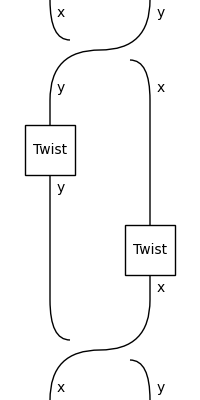# balanced#

The free balanced category, i.e. diagrams with braids and a twist.

## Summary#

 `Diagram` A balanced diagram is a braided diagram with `Twist`. `Box` A braided box is a monoidal box in a braided diagram. `Braid` Braid in a balanced category. `Twist` The twist on atomic type `dom`. `Sum` A balanced sum is a braided sum and a balanced box. `Category` A braided category is a monoidal category with a method `braid`. `Functor` A balanced functor is a braided functor that twists.

## Axioms#

The axiom for the twist holds on the nose.

```>>> x, y = Ty('x'), Ty('y')
>>> assert Diagram.twist(x @ y) == (Braid(x, y)
...     >> Twist(y) @ Twist(x) >> Braid(y, x))
>>> Diagram.twist(x @ y).draw(path="docs/_static/balanced/twist.png")
```